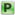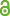Hauptmenü
• Autor
• Bazarova, Alina
• Berkes, István
• Raseta, Marko
• TitelStrong approximation of lacunary series with random gaps
• Datei
• DOI10.1007/s00605-017-1059-5
• Erschienen inMonatshefte für Mathematik
• Erscheinungsjahr2017
• LicenceCC BY
• ISSN1436-5081
• Zugriffsrechte• AbstractWe investigate the asymptotic behavior of sums $$Σ^N_{k=1} f (n_k x)$$, where f is a mean zero, smooth periodic function on $$\mathbb{R}$$ and $$(n_k )_{k≥1}$$ is a random sequence such that the gaps $$n_{k+1} − n_k$$ are i.i.d. Our result shows that, in contrast to the classical Salem-Zygmund theory, the almost sure behavior of lacunary series with random gaps can be described very precisely without any assumption on the size of the gaps.